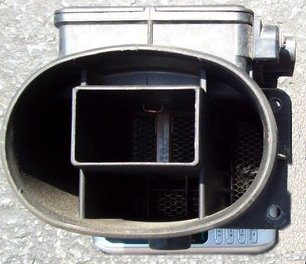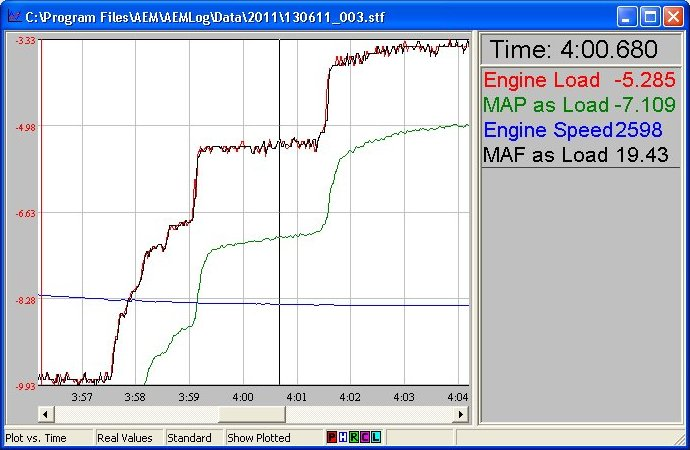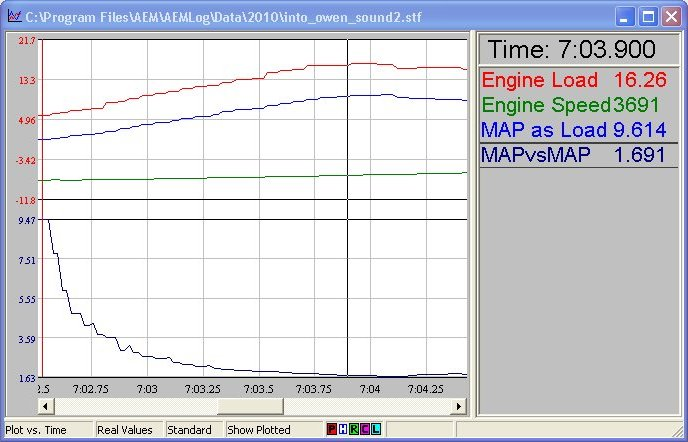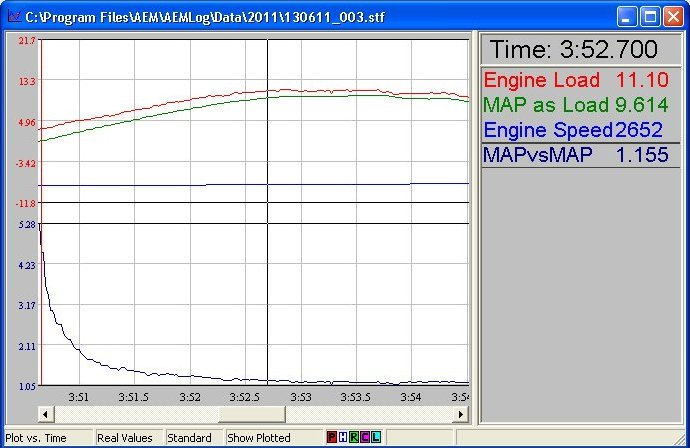### Programming the Mitsubishi MAF with the AEM EMS

Mitsubishi uses a unique Mass Airflow Sensor (MAF) in most of its EFI cars - unique, because it isn't really a real MAF. Instead, it measures air volume, not mass.This sensor uses a technique called the Karman Vortex Street to measure air volume. The inlet airstream passes over an airfoil. This airfoil generates vortices in direct proportion to the volume of air flowing over it, and a pressure transducer is used to count the vortices.

Accordingly, the output from the sensor is a pulsed (frequency) signal that is proportional to airflow volume. An Air Temperature Sensor (AIT) inside the sensor body is used in conjunction with a calibration table in order to correct volume for density.

On the MAF sensor used on a Stealth or 2G DSM, the sensor is capable of measuring roughly 2800 Hz of air, which corresponds to approximately 650 CFM.

On a Mitsubishi using a "MAF", what you really have is a volume, plus some corrections tables. This makes for a calibration which shares elements of a true Mass/Air system and a Speed/Density system - it is a hybrid of the two. You are less concerned about determining VE the way you are in a "pure" Speed/Density, because the volume sensor accounts for VE (you are measuring volume directly, not inferring it from Manifold Pressure and Engine RPM) but you still have to correct for air temp and air pressure.

That, however, is largely theoretical. You can treat the calibration as if it were a MAF-based system, once the air temp and baro pressure tables are set.

It doesn't actually scale the Load axis - don't panic! It just scales the units associated with MAF_as_Load. This is a tie-in with speed/density mode (where MAP units really are the Load axis) and strictly speaking, it's a bug. But it only affects display, not operation. Bottom line: treat Load units as a dimensionless quantity, and treat MAP_as_Load as real units, even though the software makes them look like the same thing.So then, the MAF sensor produces a frequency signal (in Hz) that corresponds to an air flow volume. The AEM transforms this frequency into a Load parameter which is then used as the Y axis in any number of tables and maps. How does that work?

Firstly, there is an Option for the MAF called VMAF_Flow_Max. This option is used to determine the maximum amount of flow that the sensor can read. Note that this is a SOFT maximum, set in software, that has NOTHING to do with the actual physical flow limit of the MAF (the point where increasing air volume no longer produces an increasing signal.

VMAF_Flow_Max works in a non-obvious way which we will describe later.

The other Option that controls the MAF is VMAF_Flow_Sum_Revs, which is used to determine over how many engine revolutions the MAF pulses are counted per "timeslice" or "window" in which we calculate load. Note that the AEM works in terms of pulses, not in terms of frequency which is how most of the systems that talk to the OEM ECUs describe airflow.

So here's the description of the AEM's operation:

1. The MAF vortex pulses (and fractional pulses) are counted in a window of engine CAMSHAFT revolutions, set in option VMAF_Flow_sum_Revs.
2. The count can be seen by logging parameter MAF_Flow_sum_raw
3. This is then corrected for barometric pressure and air temperature as shown in parameter MAF_Flow_sum_cor
4. Parameter MAF_as_Load = MAF_Flow_sum_cor / VMAF_Flow_Max as long as MAF_Flow_sum_cor in is the range defined by VMAF_to_TPS_Above to VMAF_to_TPS_Below
5. MAF_as_Load is thus a 0-100 value indicating the percentage corrected load range of the flow meter.
6. Engine Load is the same value converted to an engineering unit - which, as noted above, is scaled to the range of the MAP sensor if one is installed.
7. The scaling for humans can be adjusted via the options MAF_scalar, MAF_offset, Load_scalar, and Load_Offset (I haven't tested this yet - it might be possible to tweak MAF units to match - or at least approximate - MAP units. Or maybe it would be better to just display 1-100)
8. Parameter T4PER will indicate the period = 1/frequency of vortex meter, expressed in microseconds.

The trick is that, unlike what it says in the manual and in the Context Sensitive Help, VMAF_Flow_Sum_Revs is CAMSHAFT revs, NOT CRANK revs.

Since MAF_Flow_sum has a range of 0 to 4095.9, 6000 rpm and VMAF_Flow_sum_Revs set to "1" would give a maximum frequency of way over 100kHz. This is way too high, so the soft calibration (VMAF_Flow_Sum_Revs and VMAF_Flow_Max) has to be tweaked to produce a reasonable range of flow volume for MAF_as_Load (and subsequently Engine Load)

The AEM default values for a 3000GT are:

• VMAF_Flow_Sum_Revs = 4
• VMAF_Flow_Max = 40

So at a given engine RPM, divide by 2 to get cam revs. Then divide by VMAF_Flow_Sum_Revs to get the number of "windows" per minute in which pulses can be counted. Multiply by VMAF_Flow_Max to get pulses/min and divide by 60 to get pulses/sec (AKA Hz).

That gets you the maximum MAF frequency at that RPM that the calibration can support.

It's the at that RPM part that's so tricky. There is no timer loop in the AEM that just reads the MAF pulses and puts the current MAF frequency into a register or whatever. Instead, the program just counts MAF pulses per "window" and scales that as a 0-100% load value. (Represented as MAF_as_Load) and then further presented in engineering units (as Load)

But because the "window" gets shorter in time as the engine revs higher, the physical volume of air increases at the same MAF count at higher RPM. A count of 30 pulses in a window is less air at 3000 RPM than it is at 7000 RPM. So if VMAF_Flow_Max is too low, you run out of "flow" at a low RPM.

Here's an example:

Using VMAF_Flow_Sum_Revs = 4 and VMAF_Flow_Max = 40

```8000 RPM = 2667 Hz 7000 RPM = 2333 Hz 6000 RPM = 2000 Hz 5000 RPM = 1667 Hz 4000 RPM = 1333 Hz 3000 RPM = 1000 Hz 2000 RPM = 667 Hz 1000 RPM = 333 Hz ```

This calibration ensures that physical MAF overrun takes place at 8000 RPM - up where it is safe. But it is very easy to flow ~ 1500 Hz of air in the 4500 RPM region and hit the VMAF_Flow_Max limit. This is manifested by triggering "fuel cut" which effectively shuts the car off. (If you encounter it, you'll know - it is really brutal. You'll think you broke something!)

So if we change the value of VMAF_Flow_Max to 50, we get:

```8000 RPM = 3333 Hz 7000 RPM = 2917 Hz 6000 RPM = 2500 Hz 5000 RPM = 2083 Hz 4000 RPM = 1667 Hz 3000 RPM = 1250 Hz 2000 RPM = 833 Hz 1000 RPM = 417 Hz ```

It is now possible to physically outflow the MAF starting at 6500 RPM or so (assuming the engine will flow that much air) but we bought ~400 Hz of headspace at 5000 RPM.

Given that VE starts dropping at higher RPM, this tradeoff might be worth it.

The missing piece of the puzzle is a table that equates CFM to airflow Hz. With that, one could work out an airflow demand curve and pick the value of VMAF_Flow_Max accordingly.

By using the data from http://www.stealth316.com/2-mas_liter-per-hz.htm I was able to work out a polynomial best-fit equation to approximate the Hz to CFM data from the MAF.

y = -1E-08x3 + 2E-05x2 + 0.2452x - 2.8571 if you are curious.

With that, I could equate the max Hz the AEM EMS can read at each RPM to a CFM number. I'm not super happy with this table; I'd much rather put a MAF on a flow bench and measure it directly, but these numbers will do for now.

Then, using Engine Analyzer 2, I worked out some CFM demand values at various boost pressures. These numbers I don't trust very much, especially up top where I know errors in port size and camshaft specs and whatnot artificially choke flow - but the values returned in the midrange seem reasonable.

Plus, the calculated numbers for CFM I'm using are raw, not corrected for air temp and baro pressure. So there's about a 10% swing on the calibration side and a further 10%-ish swing on the calculated demand side.

But at 11 PSI, the default value of VMAF_Flow_Max should be good in most cases unless the air is cool-ish or dense. At 13 PSI, you're right in the middle of the error zone, and at 17 PSI, no way no how.

By changing VMAF_Flow_Max to 46 you reach the limit of the ECU to read increasing MAF frequency at 7000 RPM, meaning that above 7000 RPM, assuming the engine demand is there, it is possible to run out of headspace (something that is impossible with the default VMAF_Flow_Max) At this setting, 11 and 13 PSI are good to go with plenty of room. There is a danger spot at 17 PSI at 6000 RPM where demand is 560 CFM but the AEM can only read 540 CFM - but this is a case where the temp and pressure corrections work in our favour and it might squeak through.

And at VMAF_Flow_Max of 48, demand is always met, but now there is potential to run out of calibration headspace at 6800 RPM instead of 7000.

The bottom line here is that given my intent to run 16 PSI or so, changing VMAF_Flow_Max to 46 from 40 almost certainly buys me all the headspace I need in the MAF to keep me off the boost cut - and all I have to do to fix the fuel maps is multiply the entire table by 46/40 = 115%

So I did - and here's the result:

This is a screenshot of the pre-change relationship between Engine Load and MAP_as_Load. To help make things clearer, I created a math channel called MAP vs MAF which is simply Engine Load / MAP_as_Load. The value at 9.614 PSI MAP is roughly 1.7.And here's a similar screenshot after the change. The value at 9.614 PSI MAP roughly 1.15 - and you can see that Engine_Load has moved down the scale.Working backwards, given that the maximum value of Engine Load is 21.7, maximum MAP at max Engine Load at the default values should be 21.7 / 1.7 = 12.7 PSI (roughly what was determined by experiment) and post change it should be 21.7 / 1.15 = 18.8 PSI (roughly where the expected physical MAF overrun should be - and well above my intended max boost value of 16 PSI)

I have been driving around on this new calibration for some time, and all appears to be working. So success!